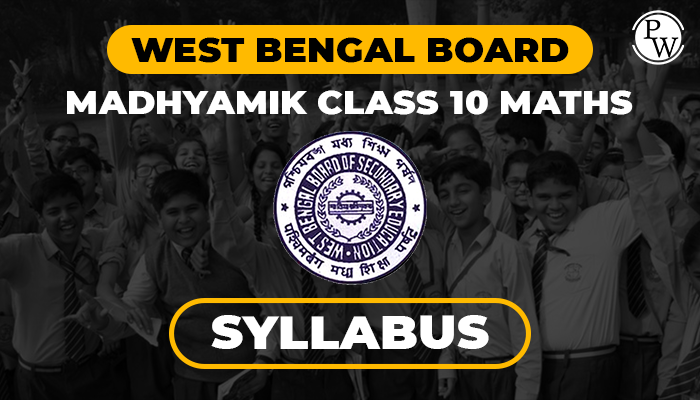# West Bengal Board Madhyamik Class 10 Maths Syllabus for Year 2022 - 23

## West Bengal Board Madhyamik Class 10 Maths Syllabus for Year 2022 - 23

WBBSE Board Madhyamik Class 10 Maths syllabus has been designed especially to satisfy the emerging needs of the scholars. Selected concepts are added to reinforce the students’ capacity or their ability to use Maths in solving lifestyle problems and at an equivalent time and motion study Maths easily.

While greater emphasis has been laid on applications of varied concepts the syllabus is additionally designed to get more interest towards the topic among students.Students also will be ready to develop better Mathematical skills. As directed by the Honorable Minister-in-Charge, School Department of Education, Government of West Bengal, the West Bengal Board of education has reduced 30-35% of the syllabus altogether the themes for Madhyamik Pariksha (S.E.), 2022-23. Students can ask the list of subject wise topics/chapters that are included for Madhyamik Pariksha – 2022-23, as given here

## Benefits of the Madhyamik Class 10 Syllabus

There are many benefits of going through the West Bengal Board Madhyamik (Class 10) syllabus. Here is a list of benifits of the West Bengal Board Class 10 syllabus:

1. Understand the topics and lessons which are included in the course material
2. Get a clear understanding and knowledge of each and every subject.
4. Be on track with the main objectives of the course.
5. Develop a correct strategy to prepare for the exam.

• Chapter 1: Quadratic equation in one variable
• Chapter 2: Simple Interest
• Chapter 3: Theorems related to circle
• Chapter 4: Rectangular Parallelopiped or Cuboid
• Chapter 5: Ratio and proportion
• Chapter 6: Compound Interest (up to 3 years) and uniform rate of increase or decrease
• Chapter 7: Theorems related to angles in a circle
• Chapter 8: Right Circular Cylinder
• Chapter 10: Theorems related to cyclic quadrilateral
• Chapter 11: Construction: Construction of circumcircle and in-circle of a triangle
• Chapter 12: Sphere
• Chapter 13: Variation
• Chapter 15: Theorems related to Tangent to a circle
• Chapter 16: Right circular cone
• Chapter 17: Construction: Construction of tangent to a circle
• Chapter 18: Similarity
• Chapter 19: Problems related to different solid objects
• Chapter 20: Trigonometry: concept of measurement of angle
• Chapter 21: Construction: Determination of mean proportional
• Chapter 22: Pythagoras theorem
• Chapter 23: Trigonometric Ratios and Trigonometric Identities
• Chapter 24: Trigonometric Ratios of complementary angles
• Chapter 25: Application of Trigonometric Ratios: Heights and Distances
• Chapter 26: Statistics: Mean, Median, Ogive, Mode

### Main Objectives of West Bengal Board Madhyamik (Class 10) Maths Syllabus

1. Develop a proper understanding of basic concepts, mathematical principles, terms etc.
2. Develop better analytical and reasoning skills.
3. Apply the acquired knowledge to resolve problems by different methods, form correct solutions and prove the results.
4. Learn about the various patterns and relationships in Mathematics.
5. Develop a robust foundation which will be useful in a student’s further study of Maths.